# SilverZone - IOM PDF Sample Papers for Class 7

Class 7 sample paper & practice questions for International Olympiad of Mathematics (IOM) level 1 are given below. Syllabus for level 1 is also mentioned for these exams. You can refer these sample paper & quiz for preparing for the exam.#### Resources:

##### Sample Questions from Olympiad Success:
 Q.1 Q.2 Q.3 Q.4 Q.5 Q.6 Q.7 Q.8 Q.9 Q.10
 Q.1 In the figure, POR is a straight line.  Find the value of a.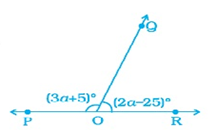a) 32° b) 55° c) 40° d) 42°
 Q.2 Name the solid with one circular face, one curved surface, and one vertex. a) Sphere b) Cylinder c) Prism d) Cone
 Q.3 Select the figure which satisfies the same conditions of placement of the dots as in figure.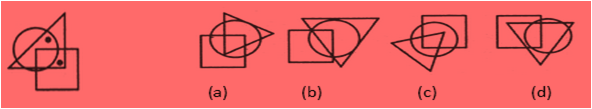a) a b) b c) c d) d
 Q.4 The bar graph given below shows the sales of books (in thousand number) from six branches of a publishing company during two consecutive years 2000 and 2001. Total sales of branch B6 for both the years is what percent of the total sales of branches B3 for both the years?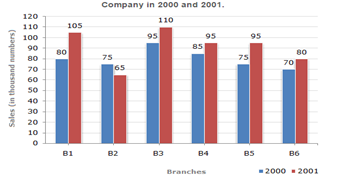a) 73.17% b) 75.55% c) 71.11% d) 68.54%
 Q.5 In the following question, find out which of the answer figures (1), (2), (3) and (4) completes the figure matrix.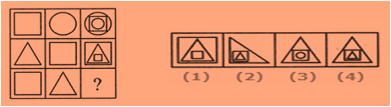a) 1 b) 2 c) 3 d) 4
 Q.6 Which of the following has the top, side and front as triangle? a)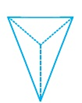b)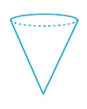c)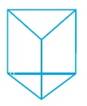d)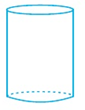Q.7 Which of the following has a line of symmetry? a)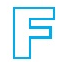b)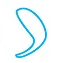c)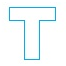d)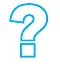Q.8 Write the usual form for 2.03 x 10-5. a) 0.203 b) 0.0000203 c) 203000 d) 0.00203
 Q.9 The sum of the ages of a football coach and his two team members is 100. His age will be the sum of the ages of the two team members in 12 years' time. How old is the football coach? a) 56 b) 28 c) 60 d) 30
 Q.10 Find the missing character from among the given alternatives.a) 25 b) 37 c) 47 d) 41Sample PDF of SilverZone - International Olympiad of Mathematics (IOM) PDF Sample Papers for Class 7:

 Q.1 )c Q.2 )d Q.3 )d Q.4 )a Q.5 )d Q.6 )a Q.7 )c Q.8 )b Q.9 )a Q.10 )d

Q.1 : c | Q.2 : d | Q.3 : d | Q.4 : a | Q.5 : d | Q.6 : a | Q.7 : c | Q.8 : b | Q.9 : a | Q.10 : d